JEE  >  Single Correct MCQs: Heat & Thermodynamics

# Single Correct MCQs: Heat & Thermodynamics - Notes | Study All Types of Questions for JEE - JEE

 1 Crore+ students have signed up on EduRev. Have you?

Q.1. In the following figure A certain mass of gas traces three paths 1, 2 and 3 from state A to state B. If the work done by the gas along these paths are W1, W2 and W3 respectively. Then-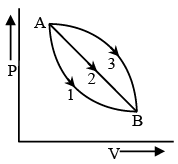(a) W1< W2< W3
(b) W1> W2> W3
(c) W1 = W2 = W3
(d) W1< W2 but W3< W
2

The work done by the gas system depends upon the area enclosed between P-V curve and volume axis.  The area enclosed by curve 3 is maximum and that enclosed by curve 1 is minimum. Hence W3> W2> W1

Q.2. At what temperature will the root mean square velocity of oxygen molecules be sufficient so as the escape from the earth?
(a) 1.6 × 105 K
(b) 16 × 105K
(c) .16 × 105 K
(d) 160 × 105

∴ 3/2 KT = 1/2 mve2
Where ve = escape velocity of earth = 11.1 km/sec
m = mass of 1 molecule of oxygen = 5.34 × 10–26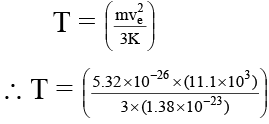⇒ T = 1.6 × 105 K

Q.3. In the following indicator diagram (Figure), the net amount of work done will be -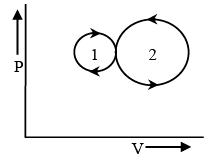(a) Positive
(b) negative
(c) Zero
(d) infinity

The cyclic process 1 is clockwise and the process 2 is anticlockwise.  Therefore W, will be positive and W2 will be negative. Area 2 > area 1, Hence the network will be negative.

Q.3. In the following figure two indicator diagrams are shown.  If the amounts of work done in them are W1 and W2 respectively, then –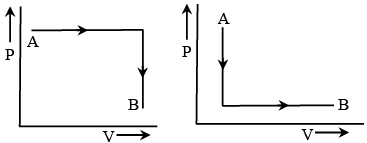(a) W1> W2
(b) W1< W2

(c) W= W2
(d) W1 = W2/4

The area enclosed by first P-V curve is greater than that of by the second curve, Therefore, W1> W2.

Q.4. The first excited state of hydrogen atom is 10.2 eV above its ground state. What temperature is needed to excite hydrogen atoms to first excited level –
(a) 7.88 × 10K
(b) .788 × 104

(c) 78.8 × 104 K
(d) 788 × 104

K.E. Per atom = 3/2 KT
K.E. of the hydrogen atom = 10.2 eV
10.2 eV = 10.2 × (1.6 × 10–19) Joule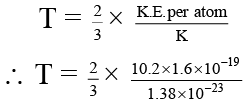Where, k = 1.38 × 10–23 J/mole. ºK
⇒ T = 7.88 × 104 K

Q.6. The atomic weight of iodine is 127. A standing wave in a tube filled with iodine gas at 400 K has nodes that are 6.77cm apart when the frequency is 1000 vib/sec. iodine is
(a) Monoatomic
(b) Diatomic
(c) Triatomic
(d) None of these

∴ λ = 2 × 6.77 cm = 13. 54cm
v = nλ = 1000 × 13.54 = 1.354 × 104cm/sec.

We know that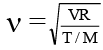where molecular weight
M = Ax with x = 1 if iodine is monoatomic x = 2 it diatomic and A is atomic weight
∴ γ = Axv2/RT = 0.7
Where x = 2 as iodine is diatomic
∴ γ = 1.4 (right value of diatomic gas)

Q.7. A block of ice of mass 50 Kg. is pushed out on a horizontal plane with a velocity of 5 M/s. Due to friction it comes to rest after covering a distance of 25 meter. How much ice will melt?
(a) 0.86 gm
(b) 1.86 gm
(c) 100 gm
(d) 1000 gm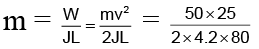m = 1.86 gm.

Q.8. M kilograms of a material are to be kept in melted state at melting point and power required for this purpose is P.  When the power source is disconnected then the sample completely solidifies in t second.  The latent heat of fusion of the material will be -
(a) M/Pt
(b) Pt/M
(c) PtM
(d) Pt

Heat released in t second Q = ML ...(A)
Loss of heat per second  P =  Q/t ...(B)

From equation (A) and (B) P = ML/t
∴ L = Pt/M

Q.9. Isothermal curves are obtained by drawing –
(a) P against V
(b) P against T
(c) PV against R
(d) PV against V

In an isothermal process, temperature remains constant and process equation is, PV = constant So a graph is drawn between P and V.

Q.10. dU = – dW is true for –
(a) Isothermal process
(c) Isobaric process
(d) Isochoric process

In an adiabatic process, heat cannot be exchanged between the system and surrounding so,
dQ = 0
By Ist law of thermodynamics
dQ = dU + dW
dU= –dW

Q.11. In a certain process the pressure of one mole ideal gas varies with volume according to the relation P =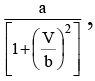where a, b are constants, when the volume of gas V = b, then temperature of the gas will be -
(a) ab
(b) ab/R
(c) ab
(d) zero

∴ T = PV/R

At V = b, P = a/(1 + 1) = a/2
∴ ab/ 2R

Q.12. The internal energy of monatomic and diatomic gases are respectively due to
(a) Linear motion and rolling motion
(b) Rolling motion and linear motion
(c) Linear motion and rotatory motion
(d) Rotatory motion and linear motion

The internal energy of a monatomic gas is due to linear motion only and that of the diatomic gas is due to rolling (linear rotatory) motion.

Q.13. In the figure (A) indicator diagram, the net amount of work done will be :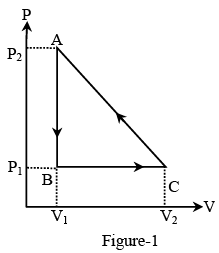(a) Positive
(b) Negative
(c) Zero
(d) Infinity

The cyclic process 1 is clockwise and the process 2 is anticlockwise. Therefore w1 will be positive and w2 will be negative area2> area1. Hence, the network will be negative.

Q.14. The pressure of an ideal gas is written as P =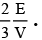Here E refers to –
(a) Translational kinetic energy
(b) Rotational kinetic energy
(c) Vibrational kinetic energy
(d) Total kinetic energy

Pressure of the gas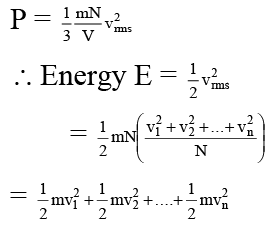So energy is basically the sum of energies of all molecules which represents translational kinetic energy.

Q.15. Which of the following parameters is the same for molecules of all gases at a given temperature?
(a) Mass
(b) Speed
(c) Momentum
(d) Kinetic energy

Kinetic energy according to kinetic theory of gases is given by,
E = 3/2 RT
R is a universal constant and temperature remains same for all gases. So kinetic energy is same for molecules of all gases at a given temperature.

Q.16. In the above question find out heat given to the system or taken out from the system in the process C → A and network done in complete cycle.
(a) – 200J, 50J
(b) – 200J, 60J
(c) 60J, – 200J
(d) +200J , – 69J

For the process C → A, ΔU = UA - U= 0 - 80
⇒ ΔU = - 80J

and W = area ACED = area ACB + area ABED
∴ W = ( 1/2 × AB × BC) + (DE × DA)

∴ W = (1/2 × 2 × 60) + ( 2 × 30) = 120 J

Since, in this process the volume decreases, the work will be negative (w = 120Joule). That is, the work will be done on the system. Now, by the first law of thermodynamics, will have
Q = ΔU + W = - 80 - 120 = - 200J
Since it is negative, this heat is given out by the system and work done in the whole cycle = area ABC =  1/2 × 2 × 60 = 60J
Since, the cyclic process is traced anticlowise, the net work will be done on the system.

Q.17. A Carnot engine takes 103 kilocalories of heat from a reservoir at 627ºC and exhausts it to a sink at 27ºC. The efficiency of the engine will be
(a) 22.2%
(b) 33.3%
(c) 44.4%
(d) 66.6%

Efficiency of Carnot engine
η = 1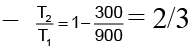∴ η = 66.6 %

Q.18. From the relation Cp-Cv =  R/J it is inferred that
(a) the gas is monatomic
(b) gas is diatomic
(c) gas obeys ideal gas equation irrespective of whether it is mono or diatomic
(d) gas is monatomic and it can be ideal or real

Gas obeys ideal gas equation irrespective of whether it is mono or diatomic.

Q.19. The ratio of number of collisions per second at the walls of containers by H2 and Ne gas molecules kept at same volume and temperature is –
(a) 10 : 1
(b) 1 : 10
(c) 1 : √10
(d) √10 : 1

Let's consider the wall perpendiculars to x-axis number of collisions per second are given by vx/2L Now, vx = vrms/3

Q.20. In a given process on an ideal gas dW = 0 and dQ < 0, then for the gas
(a) the temperature will decreases.
(b) the volume will increases.
(c) the pressure will remain constant.
(d) the temperature will increase

dQ < 0
dU < 0 (or dW = 0)
T2 < T1
Hence, (a) is correct.

The document Single Correct MCQs: Heat & Thermodynamics - Notes | Study All Types of Questions for JEE - JEE is a part of the JEE Course All Types of Questions for JEE.
All you need of JEE at this link: JEE

## All Types of Questions for JEE

376 docs
 Use Code STAYHOME200 and get INR 200 additional OFF

## All Types of Questions for JEE

376 docs

Track your progress, build streaks, highlight & save important lessons and more!

,

,

,

,

,

,

,

,

,

,

,

,

,

,

,

,

,

,

,

,

,

;